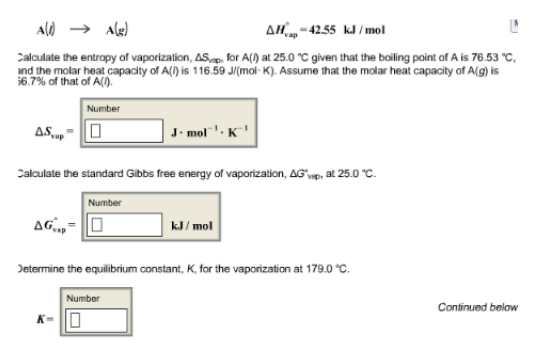# Problem: A (l) → A (g)                       ΔH°vap = -42.55 kJ/mol Calculate the entropy of vaporization, ΔSvap, for A(/) at 250°C given that the boiling point of A is 76.53°C, and the molar heat capacity of A() is 116.59 J/(mol K). Assume that the molar heat capacity of A(g) is 6.7% of that of A(l). Calculate the standard Gibbs free energy of vaporization, ΔG°vap, at 25.0°C. Determine the equilibrium constant, K, for the vaporization at 179.0°C.

###### FREE Expert Solution
100% (208 ratings)###### Problem Details

A (l) → A (g)                       ΔH°vap = -42.55 kJ/mol

Calculate the entropy of vaporization, ΔSvap, for A(/) at 250°C given that the boiling point of A is 76.53°C, and the molar heat capacity of A() is 116.59 J/(mol K). Assume that the molar heat capacity of A(g) is 6.7% of that of A(l).

Calculate the standard Gibbs free energy of vaporization, ΔG°vap, at 25.0°C.

Determine the equilibrium constant, K, for the vaporization at 179.0°C.